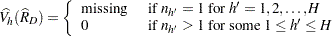### Domain Statistics

When you use a DOMAIN statement to request a domain analysis, the procedure computes the requested statistics for each domain.

For a domain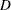, let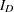be the corresponding indicator variable: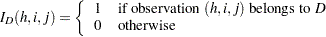Let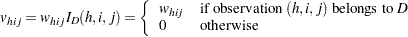The requested statistics for variable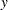in domainare computed by using the new weights.

#### Domain Mean

The estimated mean ofin the domainis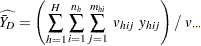where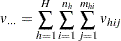The variance of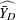is estimated by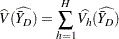where if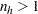,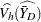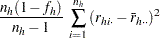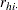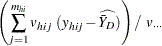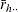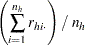and if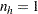,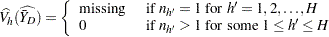#### Domain Total

The estimated total in domainis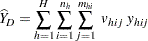and its estimated variance is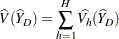where if,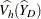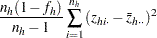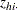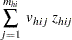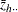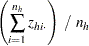and if,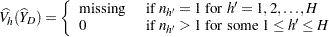#### Domain Ratio

The estimated ratio of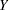to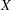in domainis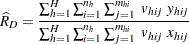and its estimated variance is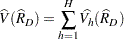where if,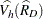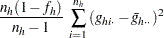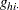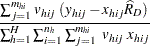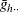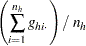and if,